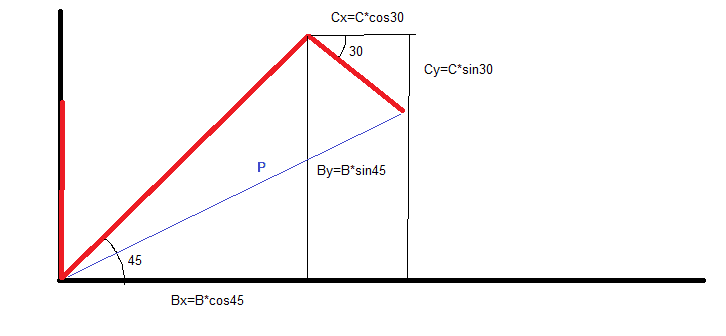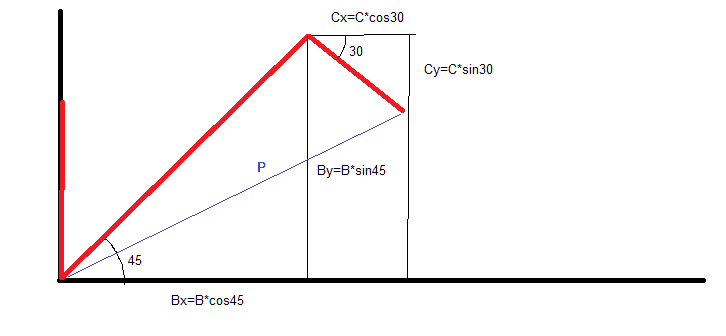# Help with Vector Addition Problem

• Josh Eiland

## Homework Statement

In photo:
https://gyazo.com/6348b5ffe368852ee9868a2ba0dd7e38

In image

## The Attempt at a Solution

In the boxes

Where did I go wrong with the angle calculation?

Thanks

I don't know, you didn't show the Calc.

I don't know, you didn't show the Calc.
https://gyazo.com/efc009de2080feedea420d6b2a5edab4

Triangle on the right shows how I calculated -10° angle by using tan-1(-3.2/17.4), -3.2 being the change in y and 17.4 change in x after I added the vectors.

Last edited:
I calculated the angle as -10°, but since the question asked for the angle counter-clockwise from the x axis, I thought it would be correctly expressed as 350.

-10 degrees measured from what?

-10 degrees measured from what?
From the positive x axis.

Okay where did you get the -10 degrees from?

You can find the coordinate of the player's position through your vectors. After that you can simply use geometry to determine the ccw angle, after that, 360 - positive angle = clockwise angle. The program may not want to accept negative angles, try the positive equivalent between 0 and 360.

Okay where did you get the -10 degrees from?

You can find the coordinate of the player's position through your vectors. After that you can simply use geometry to determine the ccw angle, after that, 360 - positive angle = clockwise angle. The program may not want to accept negative angles, try the positive equivalent between 0 and 360.

https://gyazo.com/efc009de2080feedea420d6b2a5edab4

Triangle on the right shows how I calculated -10° angle by using tan-1(-3.2/17.4), -3.2 being the change in y and 17.4 change in x after I added the vectors.

https://gyazo.com/efc009de2080feedea420d6b2a5edab4

Triangle on the right shows how I calculated -10° angle by using tan-1(-3.2/17.4), -3.2 being the change in y and 17.4 change in x after I added the vectors.

And yes, that's how I got 350° counter-clockwise. By doing 360 minus the clockwise angle (360-10).

From the positive x axis.

I think if you draw yourself a picture you will see that it can't be -10 degrees from the +ve x-axis.

I think if you draw yourself a picture you will see that it can't be -10 degrees from the +ve x-axis.
I got -10 from the X-axis counter-clockwise when drawing a picture, as I've explained. Do you have a better answer or suggestion?

I could not understand your drawings. So my suggestion is to redraw a simple picture that summarizes the vectors and shows the -10 degrees.From this image, find the vector P.From this image, find the vector P.
That wouldn't make sense, as the angle from initial to final points would be from the top of the red line on the y-axis to the final spot, not from the origin.

I could not understand your drawings. So my suggestion is to redraw a simple picture that summarizes the vectors and shows the -10 degrees.
I calculated the final point (17.4, -3.2) and drew a line from the origin (0,0) to this point, creating a triangle with the x and y segments. Using Pythagorean theorem I calculated the magnitude of this vector 17.7
I then used inverse tangent to find the angle -10°

Ok, I follow you now. I think you got it right. Maybe a small rounding error. Try and see if 349.6 works.

Ok, I follow you now. I think you got it right. Maybe a small rounding error. Try and see if 349.6 works.
Tried it, didn't work. I think the teacher probably just put the answer in wrong accidentally. Will ask tomorrow. Thanks for help.

That is not the answer that I am getting at all.

I am getting a positive angle of 24.213 degrees counter-clockwise.

Again, if you're not showing your calculations it's impossible for us to tell you where you're going wrong.

Bx=11.3137
By=11.3137
Cx=6.0622
Cy=3.5

Therefore the resultant vector is
r=[Bx+Cx,By-Cy]

I got the same answer as the OP.

OP calculated his final Y-coordinate as a negative, it doesn't make sense from the given picture assuming that the Y-axis goes along vector A and the X-axis goes through the bottom corner.